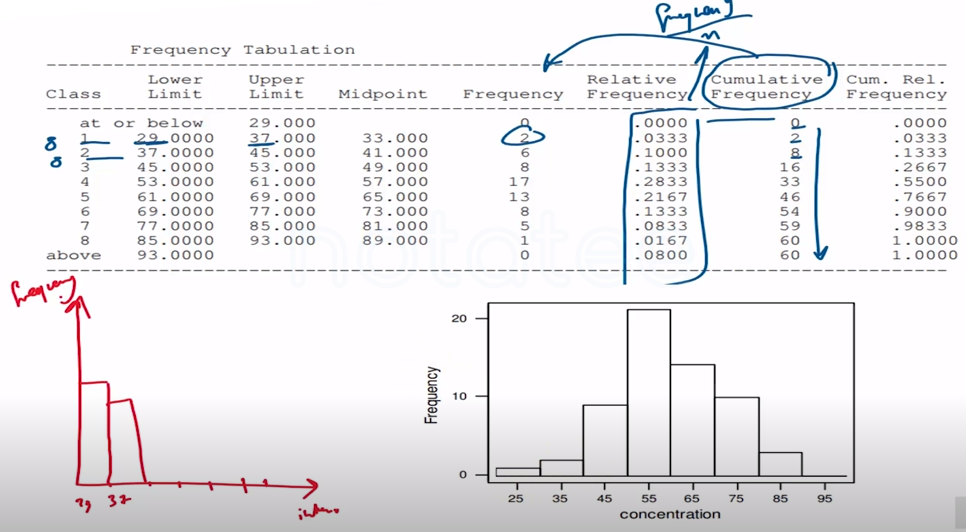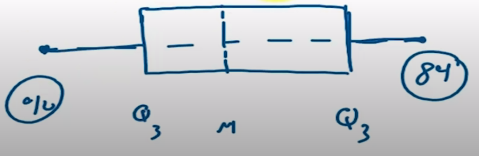Need Help?

Subscribe to Probability

###### \${selected_topic_name}
• Notes

Construct a histogram for the water quality data

$\begin{array}{llllllllll}{42.4} & {65.7} & {29.8} & {58.7} & {52.1} & {55.8} & {57.0} & {68.7} & {67.3} & {67.3} \\ {54.3} & {54.0} & {73.1} & {81.3} & {59.9} & {56.9} & {62.2} & {69.9} & {66.9} & {59.0} \\ {56.3} & {43.3} & {57.4} & {45.3} & {80.1} & {49.7} & {42.8} & {42.4} & {59.6} & {65.8} \\ {61.4} & {64.0} & {64.2} & {72.6} & {72.5} & {46.1} & {53.1} & {56.1} & {67.2} & {70.7} \\ {42.6} & {77.4} & {54.7} & {57.1} & {77.3} & {39.3} & {76.4} & {59.3} & {51.1} & {73.8} \\ {61.4} & {73.1} & {77.3} & {48.5} & {89.8} & {50.7} & {52.0} & {59.6} & {66.1} & {31.6}\end{array}$The following data are the joint temperatures of the
O-rings $^{\circ} \mathrm{F}$ ) for each test firing or actual launch of the space
shuttle rocket motor

$84,49,61,40,83,67,45,66,70,69,80,58,68,60,67,72,$
$73,70,57,63,70,78,52,67,53,67,75,61,70,81,76,79,$
$75,76,58,31$

(a) Find the upper and lower quartiles of temperature.
(b) Find the median.
(c) Construct a box plot of the data and comment on the pos-
sible presence of outliers.

Q1 , Q2 , Median

$40 \longrightarrow 84$

$Q_{1}=58.5$
$Q_{3}=75.00$

Median $=67.50$